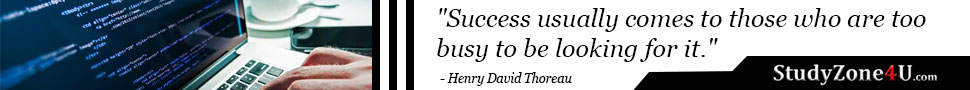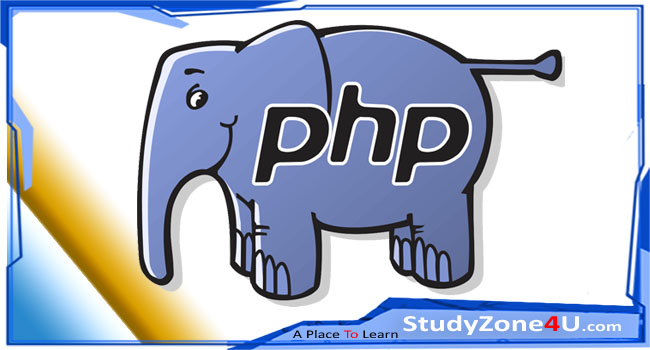# Converting array of objects to array in php

Last updated 4 years, 5 months ago | 1295 views

Tags:- PHP# PHP | Converting array of objects to array

Array of objects look like this:

``````Array
(
 => stdClass Object
(
[id] => 1
[name] => Ram
[roll] => 12
)
 => stdClass Object
(
[id] => 2
[name] => Shyam
[roll] => 36
)
 => stdClass Object
(
[id] => 6
[name] => Mohan
[roll] => 24
)
)``````

Here we can see how to convert an array of objects into an array. We can use type casting for the same. Typecasting is a way to use/convert one data type variable into the other data type. This can be done with a single line of code.

Actually, these object comes from \$object variable.

So, for typecasting, write the name of the desired type in parentheses before the variable which is to be cast.

In this post we are converting the records in an array. so we write (array) in parentheses.

``````\$array = (array) \$object;

print_r(\$array);``````

we can achieve the same using json_encode and json_decode function which always live in PHP.

Here the code for the same:

``````\$json  = json_encode(\$object);
\$array = json_decode(\$json, true);

print_r(\$array);``````